# Expression Operators

#### Fabrica Bolos GLPK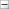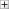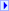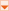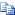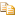#Operators

NameDescription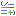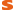Addition(Double, Expression)
Adds a constant to an expressionAddition(Expression, Expression)
Implements the operator +.Addition(Expression, Variable)
Implements the operator +.Addition(Expression, Double)
Adds a constant to an expressionAddition(Variable, Expression)
Implements the operator +.Equality(Expression, Expression)
Implements the operator ==.Equality(Expression, Variable)Equality(Expression, Double)
Implements the operator ==.Equality(Variable, Expression)
Creates a new equality constraintGreaterThanOrEqual(Double, Expression)
Implements the operator >=.GreaterThanOrEqual(Expression, Expression)
Implements the operator >=.GreaterThanOrEqual(Expression, Variable)
Implements the operator >=.GreaterThanOrEqual(Expression, Double)
Implements the operator >=.GreaterThanOrEqual(Variable, Expression)
Implements the operator >=.Inequality(Expression, Expression)
Please construct inequalities using <= and >=Inequality(Expression, Variable)
Please construct inequalities using <= and >=Inequality(Expression, Double)
Please construct inequalities using <= and >=Inequality(Variable, Expression)
Please construct inequalities using <= and >=LessThanOrEqual(Double, Expression)
Implements the operator <=.LessThanOrEqual(Expression, Expression)
Implements the operator <=.LessThanOrEqual(Expression, Variable)
Implements the operator <=.LessThanOrEqual(Expression, Double)
Implements the operator <=.LessThanOrEqual(Variable, Expression)
Implements the operator <=.Multiply(Double, Expression)
Implements the operator *.Multiply(Expression, Expression)
Implements the operator *.Multiply(Expression, Double)
Implements the operator *.Subtraction(Double, Expression)
Implements the operator -.Subtraction(Expression, Expression)
Implements the operator -.Subtraction(Expression, Variable)
Implements the operator -.Subtraction(Expression, Double)
Implements the operator -.Subtraction(Variable, Expression)
Implements the operator -.